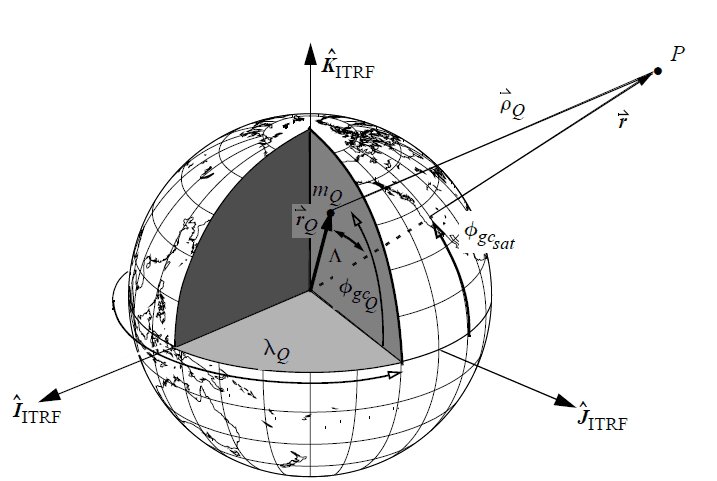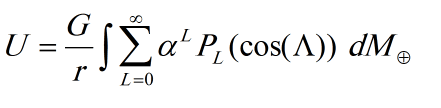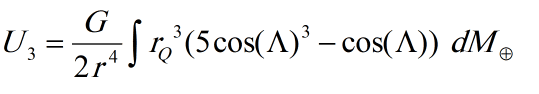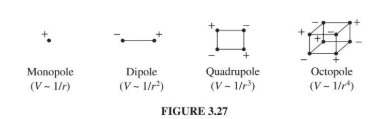# A non-spherical Earth's gravitational potential?

patric44
hi guys
i was reading a book on astrodynamics and was trying to understand the mathematical treatment of the Earth gravitational potential . i kinda understand the main idea , after reaching the following equation of the potential in terms of the Legendre polynomials :##\alpha = r_{Q}/r##

i am really interested in the geometrical interpretation of this equation which is known as MacCullagh’s method , the 1st , 2nd and the third expansion terms was some how understandable in which they some how determine the moment of inertia and different mass distribution contribution to the non sphereicity , but i am curious abut the 4th term :it some how seems like we start the series with the regular spherical potential and each successive term held some kind a contribution to the non sphericity so every term should have a physical meaning , right! , what this term mean is it also some kind of a fancy combination of moment of inertial around some point or something ?!

•etotheipi and Delta2

mpresic3
Every term in the harmonic expansion reflects more "fine structure" regarding the shape of the Earth, but also the density distribution of the Earth as well. For example, Mt Everest alone is pretty local, as far as the whole Earth is concerned, but Mt Everest alone would contribute to higher degree harmonics. I think the number of harmonics in some Earth models is up to order 2190, with over 4 million coefficients, with higher order models to come. These coefficients for these harmonics are obtained by fitting local gravity observations throughout the entre world. Geodesists realize it is not fruitful to characterize the harmonics based on "what would be expected physically", based on the order. There are just too many coefficients.

For more detail Heiskanen and Moritz is sort of the bible among geodesists.

••etotheipi, vanhees71 and patric44
mpresic3
If I may add a more satisfactory answer to your question. The zero-th degree is the spherical monopole expansion in the gravitational potential. The first degree term is the dipole expansion. The second degree term is the quadrupole term and related to the moments of inertia which are integral r squared dm's, the third degree term is (spherical) octopole moments, etc

•patric44
patric44
thanks so much for the explanation , i have a little question : how the expansion terms mathematically knows that there is something on the Earth that might cause that harmonic?! , or am i missing something , i am sorry i don't really get what you mean by "octopole moment" can you explain it a little bit 😅

Homework Helper
Gold Member
It might be worth considering multipole moments in electrostatics.
A point charge q has a potential that falls off as 1/r.

Take two point charges q and -q, separated by a distance d, but still centered at the origin... an electric dipole.
How does the potential behave far away, at large r?
Since the net charge is zero, there is no monopole term...
This follows by superposition...
far away, the opposite sign potentials from these slightly displaced charges almost cancel.

Now take two identical dipoles and flip one and displace them slightly...
so it looks like a square centered at the origin, with alternating charges as one walks around the square.
How does the potential behave far away, at large r?
Since the net charge is zero, there is no monopole term.
The dipole term is also zero.

Keep going.

Here's a figure from Griffiths Introduction to Electrodynamics.•etotheipi, patric44 and Delta2
mpresic3
robophy covered it quite well. The real Earth does not look like one isolated charge. One charge with a dipole is a better approximation. A charge with a dipole and a quadrupole would be better still. A charge with a dipole and a quadrupole and an octopole would be even better. Ad infinitum.

In practice in Astrodynamics, it looks like you may be using Vallado, or Bate Mueller White, you do not use all 2190 terms in the latest Earth gravity model. I think (I do not know for sure) GPS uses only around 15 -20 order (perhaps 400 total coefficients) for their ephemeris. Other applications in astrodynamics do not even extend to this order.

Gold Member
2022 Award
thanks so much for the explanation , i have a little question : how the expansion terms mathematically knows that there is something on the Earth that might cause that harmonic?! , or am i missing something , i am sorry i don't really get what you mean by "octopole moment" can you explain it a little bit 😅
In textbooks you usually find this in electrodynamics texts. The good thing is you only need electrostatics. The reason is that Newtonian gravity is just a field theory with the graivtational potential ##\Phi(\vec{x})## fulfilling the Poisson equation,
$$-\Delta \Phi=-4 \pi \gamma \rho,$$
where ##\gamma## is Newton's gravitational constant and ##\rho## the mass density.

In the following we assume that there's a sphere of radius ##R## within the entire mass distribution (in this case the Earth) is located, i.e., ##\rho(\vec{x})=0## for ##r=|\vec{x}|>R##.

The general solution is as for the electrostatic Coulomb field
$$\Phi(\vec{x})=-\gamma \int_{\mathbb{R}} \mathrm{d}^3 x' \frac{\rho(\vec{x}')}{|\vec{x}-\vec{x}'|}.$$
Now the idea of the multipole expansion is to expand the corresponding Green's function of the (negative) Laplace operator in terms of spherical harmonics
$$G(\vec{x}-\vec{x}')=\frac{1}{4 \pi |\vec{x}-\vec{x}'|}=\sum_{\ell=0}^{\infty} \frac{r_{<}^{\ell}}{(2 \ell+1)r_{>}^{\ell+1}} \sum_{m=-\ell}^{\ell} \text{Y}_{\ell m}^*(\vartheta',\varphi') \text{Y}_{\ell m}(\vartheta,\varphi),$$
Here ##(r,\vartheta,\varphi)## and ##(r',\vartheta',\varphi')## are the spherical coordinates of ##\vec{x}## and ##\vec{x}'##, respectively and ##r_{>}=\text{max}(r,r')## and ##r_{<}=\text{min}(r,r')##.

Plugging this into the solution you get
$$\Phi(\vec{x})=-\gamma \sum_{\ell=0}^{\infty} \sum_{m=-\ell}^{\ell} \frac{P_{\ell m}}{r^{\ell}} \text{Y}_{\ell m}(\vartheta,\varphi)$$
with the multipole moments
$$P_{\ell m}=\int_{0}^{R} \mathrm{d} r' \int_0^{\pi} \mathrm{d} \vartheta \int_0^{2 \pi} \frac{4 \pi}{2 \ell+1} r^{\prime \ell+2} \text{Y}_{\ell m}^{*}(\vartheta,\varphi) \rho(\vec{r}').$$

•etotheipi